# NCERT Solutions for Class 8 Maths Exercise 6.1## myCBSEguide App

CBSE, NCERT, JEE Main, NEET-UG, NDA, Exam Papers, Question Bank, NCERT Solutions, Exemplars, Revision Notes, Free Videos, MCQ Tests & more.

NCERT Solutions for Class 8 Maths Exercise 6.1 Class 8 Maths book solutions are available in PDF format for free download. These ncert book chapter wise questions and answers are very helpful for CBSE exam. CBSE recommends NCERT books and most of the questions in CBSE exam are asked from NCERT text books. Class 8 Maths chapter wise NCERT solution for Maths Book all the chapters can be downloaded from our website and myCBSEguide mobile app for free.

NCERT solutions for Class 8 Maths Squares and Square Roots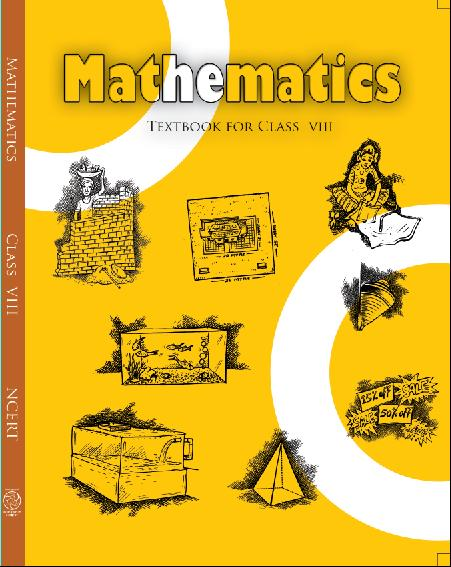## NCERT Solutions for Class 8 Maths Squares and Square Roots

###### 1. What will be the unit digit of the squares of the following numbers:

(i) 81

(ii) 272

(iii) 799

(iv) 3853

(v) 1234

(vi) 26387

(vii) 52698

(viii) 99880

(ix) 12796

(x) 55555

Ans. (i) The number 81 contains its unit’s place digit 1. So, square of 1 is 1.

Hence, unit’s digit of square of 81 is 1.

(ii) The number 272 contains its unit’s place digit 2. So, square of 2 is 4.

Hence, unit’s digit of square of 272 is 4.

(iii) The number 799 contains its unit’s place digit 9. So, square of 9 is 81.

Hence, unit’s digit of square of 799 is 1.

(iv) The number 3853 contains its unit’s place digit 3. So, square of 3 is 9.

Hence, unit’s digit of square of 3853 is 9.

(v) The number 1234 contains its unit’s place digit 4. So, square of 4 is 16.

Hence, unit’s digit of square of 1234 is 6.

(vi) The number 26387 contains its unit’s place digit 7. So, square of 7 is 49.

Hence, unit’s digit of square of 26387 is 9.

(vii) The number 52698 contains its unit’s place digit 8. So, square of 8 is 64.

Hence, unit’s digit of square of 52698 is 4.

(viii) The number 99880 contains its unit’s place digit 0. So, square of 0 is 0.

Hence, unit’s digit of square of 99880 is 0.

(ix) The number 12796 contains its unit’s place digit 6. So, square of 6 is 36.

Hence, unit’s digit of square of 12796 is 6.

(x) The number 55555 contains its unit’s place digit 5. So, square of 5 is 25.

Hence, unit’s digit of square of 55555 is 5.

###### 2. The following numbers are obviously not perfect squares. Give reasons.

(i) 1057

(ii) 23453

(iii) 7928

(iv) 222222

(v) 64000

(vi) 89722

(vii) 222000

(viii) 505050

Ans. (i) Since, perfect square numbers contain their unit’s place digit 1, 4, 5, 6, 9 and even numbers of 0.

Therefore 1057 is not a perfect square because its unit’s place digit is 7.

(ii) Since, perfect square numbers contain their unit’s place digit 0, 1, 4, 5, 6, 9 and even number of 0. Therefore 23453 is not a perfect square because its unit’s place digit is 3.

(iii) Since, perfect square numbers contain their unit’s place digit 0, 1, 4, 5, 6, 9 and even number of 0. Therefore 7928 is not a perfect square because its unit’s place digit is 8.

(iv) Since, perfect square numbers contain their unit’s place digit 0, 1, 4, 5, 6, 9 and even number of 0. Therefore 222222 is not a perfect square because its unit’s place digit is 2.

(v) Since, perfect square numbers contain their unit’s place digit 0, 1, 4, 5, 6, 9 and even number of 0. Therefore 64000 is not a perfect square because its unit’s place digit is single 0.

(vi) Since, perfect square numbers contain their unit’s place digit 0, 1, 4, 5, 6, 9 and even number of 0. Therefore 89722 is not a perfect square because its unit’s place digit is 2.

(vii) Since, perfect square numbers contain their unit’s place digit 0, 1, 4, 5, 6, 9 and even number of 0. Therefore 222000 is not a perfect square because its unit’s place digit is triple 0.

(viii) Since, perfect square numbers contain their unit’s place digit 0, 1, 4, 5, 6, 9 and even number of 0. Therefore 505050 is not a perfect square because its unit’s place digit is 0.

###### 3. The squares of which of the following would be odd number:

(i) 431

(ii) 2826

(iii) 7779

(iv) 82004

Ans. (i) 431 – Unit’s digit of given number is 1 and square of 1 is 1. Therefore, square of 431 would be an odd number.

(ii) 2826 – Unit’s digit of given number is 6 and square of 6 is 36. Therefore, square of 2826 would not be an odd number.

(iii) 7779 – Unit’s digit of given number is 9 and square of 9 is 81. Therefore, square of 7779 would be an odd number.

(iv) 82004 – Unit’s digit of given number is 4 and square of 4 is 16. Therefore, square of 82004 would not be an odd number.

NCERT Solutions for Class 8 Maths Exercise 6.1

###### 4. Observe the following pattern and find the missing digits: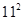= 121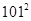= 10201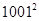= 1002001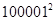= 1…….2…….1= 1……………………

Ans.= 121= 10201= 1002001= 10000200001= 100000020000001

NCERT Solutions for Class 8 Maths Exercise 6.1

###### 5. Observe the following pattern and supply the missing numbers:= 121= 10201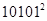= 102030201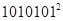= ………………………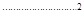= 10203040504030201

Ans.= 121= 10201= 102030201= 1020304030201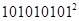= 10203040504030201

NCERT Solutions for Class 8 Maths Exercise 6.1

###### 6. Using the given pattern, find the missing numbers: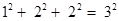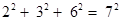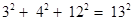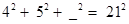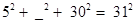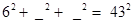Ans.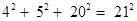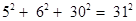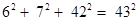NCERT Solutions for Class 8 Maths Exercise 6.1

###### 7. Without adding, find the sum:

(i) 1 + 3 + 5 + 7 + 9

(ii) 1 + 3 + 5 + 7 + 9 + 11 + 13 + 15 + 17 + 19

(iii) 1 + 3 + 5 + 7 + 9 + 11 + 13 + 15 + 17 + 19 + 21 + 23

Ans. (i) Here, there are five odd numbers. Therefore square of 5 is 25.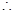1 + 3 + 5 + 7 + 9 =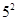= 25

(ii) Here, there are ten odd numbers. Therefore square of 10 is 100.1 + 3 + 5 + 7 + 9 + 11 + 13 + 15 + 17 + 19 =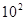= 100

(iii) Here, there are twelve odd numbers. Therefore square of 12 is 144.1 + 3 + 5 + 7 + 9 + 11 + 13 + 15 + 17 + 19 + 21 + 23 =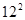= 144

NCERT Solutions for Class 8 Maths Exercise 6.1

###### 8. (i) Express 49 as the sum of 7 odd numbers.

(ii) Express 121 as the sum of 11 odd numbers.

Ans. (i) 49 is the square of 7. Therefore it is the sum of 7 odd numbers.

49 = 1 + 3 + 5 + 7 + 9 + 11 + 13

(ii) 121 is the square of 11. Therefore it is the sum of 11 odd numbers

121 = 1 + 3 + 5 + 7 + 9 + 11 + 13 + 15 + 17 + 19 + 21

NCERT Solutions for Class 8 Maths Exercise 6.1

###### 9. How many numbers lie between squares of the following numbers:

(i) 12 and 13

(ii) 25 and 26

(iii) 99 and 100

Ans. (i) Since, non-perfect square numbers between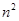and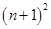are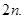Here,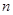= 12

Therefore, non-perfect square numbers between 12 and 13 =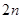=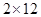= 24

(ii) Since, non-perfect square numbers betweenandareHere,= 25

Therefore, non-perfect square numbers between 25 and 26 ==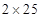= 50

(iii) Since, non-perfect square numbers betweenandareHere,= 99

Therefore, non-perfect square numbers between 99 and 100 ==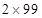= 198

## NCERT Solutions for Class 8 Maths Exercise 6.1

NCERT Solutions Class 8 Mathematics PDF (Download) Free from myCBSEguide app and myCBSEguide website. Ncert solution class 8 Mathematics includes text book solutions from Class 8 Maths Book . NCERT Solutions for CBSE Class 8 Maths have total 16 chapters. 8 Maths NCERT Solutions in PDF for free Download on our website. Ncert class 8 solutions PDF and Maths ncert class 8 PDF solutions with latest modifications and as per the latest CBSE syllabus are only available in myCBSEguide.

## CBSE app for Class 8

To download NCERT Solutions for class 8 Social Science, Computer Science, Home Science,Hindi ,English, Maths Science do check myCBSEguide app or website. myCBSEguide provides sample papers with solution, test papers for chapter-wise practice, NCERT solutions, NCERT Exemplar solutions, quick revision notes for ready reference, CBSE guess papers and CBSE important question papers. Sample Paper all are made available through the best app for CBSE students and myCBSEguide website.1. Hi